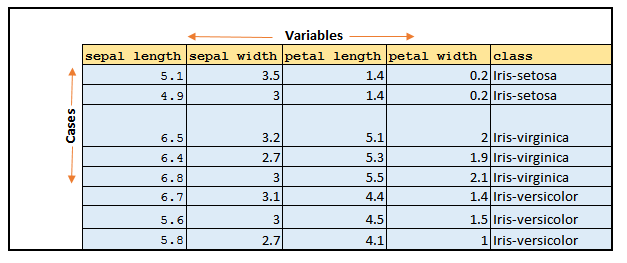### Data Matrix and Frequency table

If you’re conducting a study, you should think about your data in terms of cases and variables.

Cases are the persons, animals or things in your study, and variables are the characteristics of interest. Here, I will discuss how you can order and present your cases and variables. Lets take an example, imagine you are interested in the “Primera División”, the top football competition in Spain. Here, the cases you’re interested in are individual football players within the league, and the variables you focus on are age, body weight, goals scored, team membership and hair color. The best way to order all this information is by means of a data matrix.

So, Data Matrix is the tabular format representation of cases and variables of your statistical study. Each row of a data matrix represents a case and each column represent a variable.

A complete Data Matrix may contain thousands or lakhs or even more cases.

Sample from IRIS Dataset has shown below. You can get it from UCI Repository.

https://archive.ics.uci.edu/ml/datasets/irisTo get more insight, summarization of the information is very useful.  A good way to do that is to make a frequency table. A frequency table shows how the values of a variable are distributed over the cases. Consider this following example to consider that. We can get the frequency of items and then percentage or even calculating cumulative percentage.Here we have total 8 cases and among 8 cases 2 cases (25 % cases) belongs to Iris-Setosa.

3 cases which means 38% cases belongs to Iris-Virginia and similarly another 38% are Iris Versicolor.

Above example is for a categorical variable called class. But think if your variable is quantitative then computing percentage for every specific value does not make sense. In that case first bring your data into some ordinal categories, by using intervals. Then do the rest of the things.